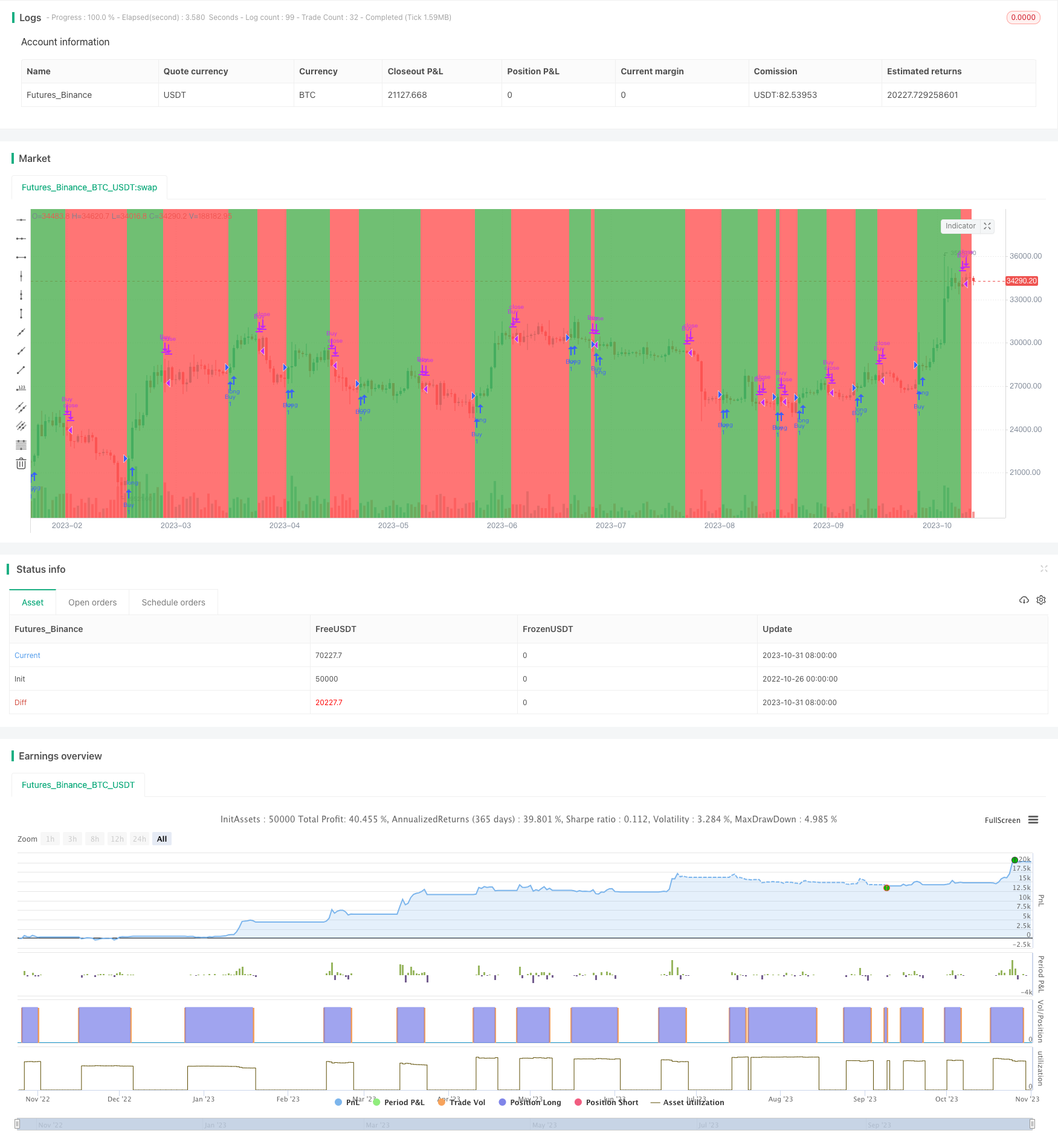# MACD Trend Prediction Strategy

Author: ChaoZhang, Date: 2023-11-02 15:25:11
Tags:## Overview

The MACD Trend Prediction Strategy is a trend following strategy based on the MACD indicator and EMA indicator. Unlike traditional MACD strategies that generate trading signals by crossing signal lines, this strategy generates trading signals by changes in the distance between the MACD line and signal line to capture trend changes.

## Strategy Logic

1. Calculate fast line DEMAfast: Calculate two EMA values of the fast line MMEfast, and calculate the fast line DEMAfast according to the formula DEMAfast = ((2 * MMEfast) - MMEfastb).

2. Calculate slow line DEMAslow: Calculate two EMA values of the slow line MMEslow, and calculate the slow line DEMAslow according to the formula DEMAslow = ((2 * MMEslow) - MMEslowb).

3. Calculate MACD line: The MACD line is the difference between fast line DEMAfast and slow line DEMAslow, LigneMACDZeroLag.

4. Calculate signal line: Calculate two EMA values of MACD line MMEsignal, and calculate signal line Lignesignal according to the formula Lignesignal = ((2 * MMEsignal) - MMEsignalb).

5. Compare MACD line and signal line: Generate buy signal when MACD line is greater than signal line, and generate sell signal when MACD line is less than signal line.

6. The above calculation uses the DEMA algorithm, which can effectively reduce the lag of MACD indicator.

1. Using the DEMA algorithm can reduce the lag of the MACD indicator and make trading signals more sensitive.

2. It does not rely on MACD crossover signals, but captures trend changes through distance changes between MACD and signal lines, which can enter trends earlier.

3. The strategy accurately judges the trend, and the profit factor can reach 1.6-3.5 with good profitability.

4. The strategy logic is simple and clear, easy to understand and implement, suitable for quantitative trading.

## Risks of the Strategy

1. As a lagging indicator, MACD may generate a lot of invalid trading signals in range-bound markets.

2. Although DEMA algorithm reduces lag, it cannot completely eliminate lag. There is still some lag.

3. As a trend following strategy, the profit may not be good in ranging markets.

4. The parameters sma, lma, tsp need to be optimized for different periods and varieties.

5. Stop loss strategies may need to be added to control losses.

## Directions for Strategy Optimization

1. Optimize sma, lma, tsp parameters to adapt to different periods and trading varieties.

2. Add dynamic stop loss strategies like ATR to control per trade loss.

3. Incorporate trend judgment indicators to avoid trading in ranging markets.

5. Optimize entry and exit logic to refine trading signal rules.

## Summary

The MACD Trend Prediction Strategy improves the calculation of MACD by using the DEMA algorithm to reduce lag, and judges the trend through distance changes between MACD and signal lines. As a trend following strategy, it can effectively capture trend changes. The profit factor can reach 1.6-3.5, with certain advantages. But it still needs further optimization of parameters, stop loss strategies, filtering ranging markets, etc. to adapt to more market environments. This will be the development direction of this strategy.

```/*backtest
start: 2022-10-26 00:00:00
end: 2023-11-01 00:00:00
period: 1d
basePeriod: 1h
exchanges: [{"eid":"Futures_Binance","currency":"BTC_USDT"}]
*/

// This source code is subject to the terms of the Mozilla Public License 2.0 at https://mozilla.org/MPL/2.0/

//@version=4
strategy("MACD Trendprediction Strategy V1", shorttitle="MACD TPS", overlay=true)
sma = input(12,title='DEMA Courte')
lma = input(26,title='DEMA Longue')
tsp = input(9,title='Signal')
dolignes = input(true,title="Lignes")

MMEslowa = ema(close,lma)
MMEslowb = ema(MMEslowa,lma)
DEMAslow = ((2 * MMEslowa) - MMEslowb )

MMEfasta = ema(close,sma)
MMEfastb = ema(MMEfasta,sma)
DEMAfast = ((2 * MMEfasta) - MMEfastb)

LigneMACDZeroLag = (DEMAfast - DEMAslow)

MMEsignala = ema(LigneMACDZeroLag, tsp)
MMEsignalb = ema(MMEsignala, tsp)
Lignesignal = ((2 * MMEsignala) - MMEsignalb )

MACDZeroLag = (LigneMACDZeroLag - Lignesignal)

bgcolor(LigneMACDZeroLag<Lignesignal ? color.red : color.green)

if (LigneMACDZeroLag>Lignesignal)